# Exercise: Vehicle powertrain modeling and cruise control

In this problem you will build a model of a vehicle in SIMULINK and control the vehicle using feedback control. The vehicle will consist of a body (chassis + wheels) and a drive train (engine + transmission). Assume that the vehicle dynamics are of the form

wherekg is the mass of the vehicle,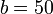N sec/m is the viscous damping coefficient, andand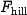represent the forces on the vehicle due to the engine and the terrain, respectively. We can implement this in SIMULINK as a two input, one output system, written in state space form aswhereis the vehicle state,is the vehicle input (two dimensional), and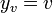is the vehicle output (velocity). You should make this into a single SIMULINK block using the "State Space" block (under SimulinkContinuousState Space} in the Simulink Library Browser). You may also want to use the Mux block (under Signals & Systems).

We will model the engine dynamics as a first order lag. Let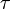represent the engine torque and assume the engine has the following dynamics:

where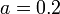is the lag coefficient,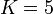is the conversion factor between engine torque and force applied to the vehicle (representing the transmission) and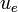is the accelerator input (which we will assume has the proper units). You should also create a SIMULINK block for this subsystem.

Finally, we include the effects of a hill. The hill simply exerts a force on the car that is based on the angle of the hill: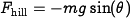wherekg m/secand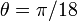is the angle of the hill (10 degrees).

1. Plot the output of the open loop vehicle model (2) for a step input of $F_\text{engine} = 500$ Newtons (assume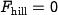). What is the rise time (0 to 95% of the final value)?

2. Plot the output of the open loop engine model for a step input of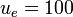Nm. What is the rise time?

3. In the homework from last week, you built a simple cruise controller. Replace the vehicle/engine model in that system with your vehicle and engine models and plot the response for the default gains ($K_i = 50$, $K_p = 1000$). Make sure to set your simulation time to be sufficiently long.

4. Now include the effect of a hill on your system. You should model the system so that the car is initially on a flat surface and then encounters the hill at $T = 100$ seconds. Plot the response of the system and compute the rise time.

Note: if you are having trouble figuring out how to create these blocks in SIMULINK, take a look at "hw1cruise.mdl" from last week's homework and see if you can modify it appropriately. It has all of the subsystems you will need (except for the Mux}block). You may also find the following web-based tutorial helpful:

http://www.engin.umich.edu/group/ctm/examples/cruise/cc.html


(ignore the sections on transfer functions; we will get to these later in the class).